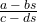# Statistics Assignment 2

Two thirds of the way through my assignments!

Again, fairly happy with the mark I received for this, but there were some aspects of this assignment I found challenging, and some where I thought I might've done quite well on, but slipped up in some way.

Let's cover some areas here:

# Finding A Real-World Process

For these questions I had to find a real-world process that could be modelled with the given mathematical objects/processes. Kind of the opposite of a mathematical modelling problem.

I found these tasks really difficult. What I found to be the worst aspect about getting this kind of question wrong is that it's not necessarily my understanding of the mathematical process that's flawed. I feel in each of these cases, I did my best to find a real world example, knowing that the example I gave, itself, was slightly flawed. So despite the fact that I can perfectly explain each mathematical process, I couldn't explain how each could be applied to a real world process so lost marks.

The two models were the Galton-Watson branching process, and the simple random walk (specifically, a particle executing a simple random walk on the line with two absorbing barriers).

The two typical examples that are referred to in my texts are genetics and mutations for the Galton-Watson branching process:

"A mutation is a spontaneous transformation of a particular gene into a different form, and this can occur by chance at any time... The mutant gene becomes the ancestor of a branching process, and geneticists are particularly interested in the probability that the mutation will eventually die out."

For the simple random walk, the example of the "gambler's ruin" was given. Imagine two people with £10 each, each of them betting on an event. If one of the two loses the bet, they give £1 to the other (the random walk on the line). If one of them runs out of money, then they lose (one of the "absorbing barriers" are hit).

In coming up with answers, I could've used Google, but that would've been cheating. However, now I've completed the assignment and received my grade, Google is my best friend in finding suitable answers here...

Seems you can use the Galton-Watson branching process to determine the extinction of a family name, and I found a good example of a random walk with absorbing barriers in this MIT paper, featuring a little flea called Stencil. It discusses the probability of him falling over the Cliff of Doom in front of him, or the Pit of Disaster behind him.

A couple of my answers here and there were classed as being incomplete. Generalising each case:

1)
Upon finding that an answer resembles a certain construction (a probability distribution function, cumulative distribution function or generating function), as well as saying which distribution the function belongs to, you should also explicitly state the variables that appear in it. Even to anyone non-mathematical, it would be obvious to see that the variables in the general case are associated with the specific answer you arrived at. Though for assignments (and exams, presumably) this is not enough. If a general function has variables explicitly state what each one is in your answer.

eg: the p.g.f. of the modified geometric distribution isIf your answer resembles this, say what a, b, c and d are.

Note that one whole mark can be deducted for an insufficient conclusion (apparently).

2)

Specifically:

To calculate the variance of the position of a particle (along the random walk line) after n steps, you can just sum the variances of each step. However this only works because each individual step is independent of the last (one of the properties of the random walk). Due to the fact that I didn't mention this definition of the variance of a particle in a random walk, I lost half a mark. Not massive, but where you can mention a definition, mention it.

# Different Routes In A Markov Chain

I struggled with this, and although I arrived at the correct answer, the method I had used was entirely wrong (and also a little inelegant).

In this question, I covered all routes separately and so had a small handful of different probability calculations. Though when considering potential routes in a Markov chain, you can consider all routes simultaneously by taking advantage of something called an absolute probability (of the Markov chain being in a particular state at a particular time), given an initial distribution. (for my own reference this is covered in Book3, Subsection 11.2, p.87. And the handbook, p.23 item 17).

# Arbitrary Constants

Does it matter if an arbitrary constant is positive or negative? (my ref: Q6a). I previously thought not. In this instance my constant in an integral calculation absorbed the negative sign that was in front if it. After all, a negative general constant is still a general constant, right? Well I lost half a mark here because of the absorption, and it's not currently clear why. I've asked my tutor, and I'll update it on here once I hear back from him.